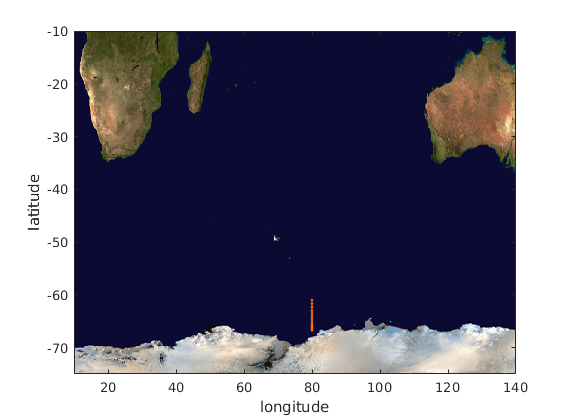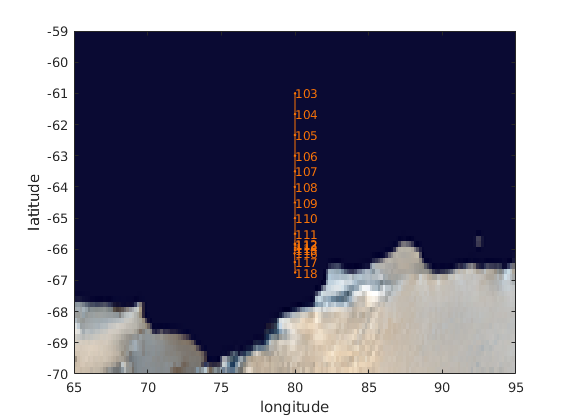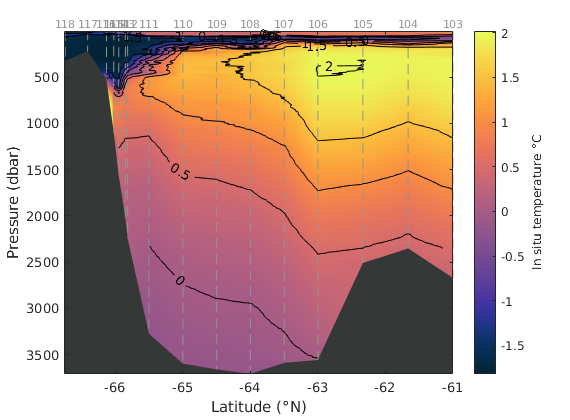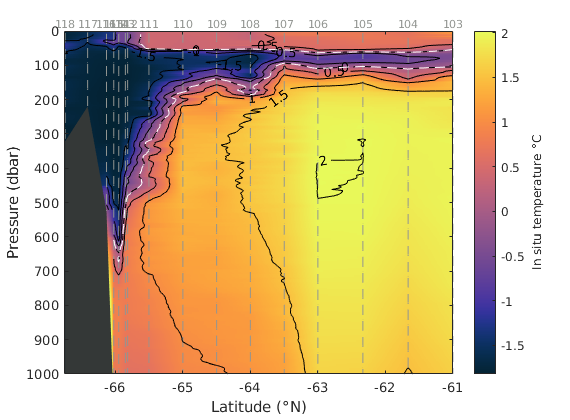# transect documentation

transect produces a color-scaled transect diagram of oceanographic data from CTD profiles collected at different locations and/or times.

## Syntax

```transect(x,d,v)
transect(...,'LineProperty',Value)
transect(...,'bed',BedDepth)
transect(...,'bedcolor',ColorSpec)
transect(...,'extrap')
transect(...,'interp','InterpMethod)
transect(...,'di',di)
transect(...,'xi',xi)
[h_trans,h_marker,h_bed] = transect(...)```

## Description

transect(x,d,v creates a color-scaled transect diagram of the variable v at the horizontal locations (or times) x and depths d. x must be a numeric array of values indicating the locations or times of each CTD profile. Inputs d and v must each be cell arrays containing depths and measurements at each CTD location. (See the section below on converting data to cell arrays.)

transect(...,'LineProperty',Value) sets the line or marker style for each datapoint. By default, each datapoint is indicated by a black dot, but profiles can just as easily be indicated with different marker types or lines of specified color, style, thickness, etc.

transect(...,'bed',BedDepth) specifies bed depths to create a patch object indicating the bed profile along the transect.

transect(...,'bedcolor',ColorSpec) specifies color of the bed. Default bedcolor is black.

transect(...,'extrap') turns on the option to extrapolate beyond the extents of data. By default, transect does not extrapolate, but the option is available to fill in gaps, such as between lowest datapoint and the seabed.

transect(...,'interp','InterpMethod) specifies an interpolation method (see the Methods section below). Default is 'linear'.

transect(...,'di',di) specifies interpolation depths di. By default, transect creates a grid by interpolating to 1000 equally-spaced depths between the shallowest and deepest measurement.

transect(...,'xi',xi) specifies interpolation locations (or times) xi. By default, transect interpolates to 2000 equally-spaced points xi between the minimum and maximum values of x.

[h_trans,h_marker,h_bed] = transect(...) returns handles of the transect plot, the markers, and the bed patch object.

## Methods

This function interpolates twice to create a gridded profile of variable v. The first round interpolates the data from each CTD profile to equally- spaced depths di. The second round interpolates horizontally to equally-spaced locations or times xi. By default, both interpolations are linear and no extrapolation is performed.

## Examples

The examples below use some Argo data that you can load like this:

```load example_ctd

whos % displays the names and sizes of each variable
```
```  Name      Size            Bytes  Class     Attributes

P         1x95            25152  cell
S         1x95            25152  cell
T         1x95            25152  cell
bed       1x95              760  double
x         1x95              760  double

```

The Argo data contain 95 profiles taken as the float drifted along. Each profile was taken at a location in x, the corresponding bed depths are given by bed and the temperatures T and salinities S are in cell arrays at the corresponding pressures P.

## Example 1: Simplest form

Here's a simple transect of temperature data T taken at locations x and depths P:

```transect(x,P,T)

xlabel 'distance along transect (km)'
ylabel 'pressure (dbar)'
```## Example 2: Specify measurement markers:

Same as above, but this time make the markers small gray dots:

```transect(x,P,T,'color',rgb('gray'),'markersize',2)

xlabel 'distance along transect (km)'
ylabel 'pressure (dbar)'
```Or instead of markers, you can make each profile its own line. Below the profiles are indicated by red dashed lines:

```transect(x,P,T,'marker','none','linestyle','--','color','r')

xlabel 'distance along transect (km)'
ylabel 'pressure (dbar)'
```## Example 4: Show the bed

To show the bed profile, just enter bed depths corresponding to each x value:

```transect(x,P,T,'bed',bed)

xlabel 'distance along transect (km)'
ylabel 'pressure (dbar)'
```Color the bed brown by specifying the RGB values of brown. If you don't know the RGB values off the top of your head, just use the rgb function:

```transect(x,P,T,'bed',bed,'bedcolor',rgb('brown'))

xlabel 'distance along transect (km)'
ylabel 'pressure (dbar)'
```## Example 5: Fill in the gaps

Don't like those gaps between the bed and the lowermost data? Or the gap near the surface between in the middle of the profile above? This function lets you extrapolate beyond the underlying data values if you wish, by specifying 'extrap':

```transect(x,P,T,'bed',bed,'extrap')

xlabel 'distance along transect (km)'
ylabel 'pressure (dbar)'

caxis([-1.9 0.9]) % sets color axis limits
```Keep in mind that it might not be appropriate to extrapolate, depending on your application. Also note that extrapolation to places below the seabed in the lower left hand corner produces some pretty high temperature values, so we had to set the color axes to match the actual oceanographic values.

## Example 6: Extend the domain

Do you want to extend the domain a little beyond the extents of the underlying data? The data go from 0 to 108 kilometers. Add 5 km on each side by specifying 'xi' values accordingly:

```% Interpolate to every 0.1 km between -5 & 113 km:
xi = -5:0.1:113;

transect(x,P,T,'extrap','xi',xi)

xlabel 'distance along transect (km)'
ylabel 'pressure (dbar)'

caxis([-1.9 0.9]) % sets color axis limits
```Be careful when extending the domain too far past the underying data. We only extrapolated a little past the data in the example above, and the edges are already a bit wonky. Try going more than 5 km and you'll really see some wacky stuff.

## Example 7: Make it fancy

Combine the options above to little gray dot markers at each measurement, include a bed profile, and set the colormap with cmocean:

```transect(x,P,T,...
'color',rgb('gray'),... % gray markers
'markersize',2,...      % small dots
'bed',bed,...           % seabed elevations
'extrap')

caxis([-1.9 0.9])          % sets color axis limits

xlabel 'distance along transect (km)'
ylabel 'pressure (dbar)'
cmocean thermal            % sets the colormap
cb = colorbar;
ylabel(cb,'temperature \circC')
```Add white contours for salinity using the transectc function:

```hold on
[C,h] = transectc(x,P,S,'w');
```Or label them:

```delete(h) % deletes contours from above

[C,h] = transectc(x,P,S,'w','ShowText','on','LabelSpacing',1000);
```Add your favorite salinity contour and make it pea green:

```[C,h] = transectc(x,P,S,[34.5 34.5]);

h.Color = rgb('pea green');
h.LineWidth = 3;
``````clear % clears previous data before the following example
```

## Converting ODV text data into a cell array

For this example, we'll use cruise some data that has been saved as a .txt file by Ocean Data Viewer. The data provided with CDT are a subset of oceanographic profile data from a 2005/2005 cruise off the coast of Antarctica. The full dataset is described on the Australian Antarctic Data Centre's website here, but we'll just use the subset that comes with CDT as an example file.

```D = importdata('BROKE_cruise_odv.txt')
```
```D =
struct with fields:

data: [9220×7 double]
textdata: {'Stn'  'Lat'  'Lon'  'Pr'  'T'  'S'  'O2'}
colheaders: {'Stn'  'Lat'  'Lon'  'Pr'  'T'  'S'  'O2'}
```

The data field contains seven columns, corresponding to:

1. station number
2. latitude
3. longitude
4. pressure
5. temperature
6. salinity
7. oxygen

If you open up the BROKE_cruise_odv.txt file, you'll see that the first column contains the number 103, repeated 668 times. That's because station 103 contains 668 temperature, salinity, and oxygen measurements, each at a different pressure (depth). Below the 668 rows of data corresponding to station 103, there are 584 rows corresponding to station 104, and so on. In total, there are 16 unique stations, each with a different number of rows of data.

Create a 16x1 stn array containing the 16 unique station numbers along with the row indices which will allow us to get the locations of those stations:

```% Unique station numbers:
[stn,ind] = unique(D.data(:,1));
```

With the indices ind corresponding to each station, get the 16 locations of each station like this, because latitude and longitude are the second and third columns of D.data:

```lat = D.data(ind,2);
lon = D.data(ind,3);
```

Here's the greographic track of the ship:

```figure
axis([10 140 -75 -10]) % sets axis limits
earthimage
hold on
plot(lon,lat,'.-','color',rgb('orange'))
xlabel 'longitude'
ylabel 'latitude'
```Zoom in on the figure to see that each CTD station is numbered 103 through 118.

```text(lon,lat,num2str(stn),... % labels each station
'fontsize',9,...
'color',rgb('orange'))

axis([65 95 -70 -59]) % sets axis limits
```To to put all the measurements into cell arrays, loop through each unique station, finding all the row indicies associated with each station number, like this:

```for k = 1:16

% Get indices of station corresponding to stn(k):
ind = D.data(:,1)==stn(k);

% Put pressure, temperature, etc of this station into cell arrays:
Pr{k} = D.data(ind,4);
T{k} = D.data(ind,5);
S{k} = D.data(ind,6);
O2{k} = D.data(ind,7);
end
```

Now T{1} contains all the temperature measurements for the CTD cast at

```stn(1)
```
```ans =
103
```

...station number 103, and Pr{1} contains the corresponding pressures.

## Plot a single cast

Cell arrays allow data of diffent sizes to be stored within a single structure, and that's useful when each CTD cast has a different number of measurements, taken at different depths.

Given that each cell contains the data from a unique CTD cast, plotting a single cast is relatively straightforward. Here we plot the data from station 110 (stn(8)). Temperature is plotted on the first (bottom) axis and salinity is plotted on the second (top) axis.

```figure
plot(T{8},Pr{8},'color',rgb('deep blue'))
ax1 = gca;
set(ax1,'XColor',rgb('deep blue'),...
'YDir','reverse');
xlabel('In situ Temperature')
ylabel('Pressure (dbar)')

ax1_pos = ax1.Position; % store the position of the first axis.
ax2 = axes('Position',ax1_pos,...
'XAxisLocation','top',...
'YAxisLocation','right',...
'Color','none',...
'XColor',rgb('deep red'),...
'YTickLabel',[],...
'YDir','reverse');
hold on
plot(S{8},Pr{8},'Parent',ax2,'color',rgb('pale red'))
grid on
xlabel('Salinity')
```## Plot multiple casts with the transect function

Take the data that is now stored in cell arrays and plot a transect of the temperature and salinity data. Assuming each CTD cast hit the bottom, we can use the maximum pressure at each station as the bed depth:

```% Assume max pressure is bed depth:
maxPress = cellfun(@max,Pr);

figure
transect(lat,Pr,T,...
'color',rgb('gray'),...
'marker','none',...
'linestyle','--',...
'bed',maxPress,...
'extrap',...
'bedcolor',rgb('charcoal'));

caxis([-1.82 2.02])

cmocean('thermal')
cb = colorbar;
ylabel(cb,'In situ temperature \circC')
xlabel 'Latitude (\circN)'
ylabel 'Pressure (dbar)'

% Label the stations:
text(lat,zeros(size(lat)),num2str(stn),...
'vert','bottom',... % pins text labels from their bottoms
'horiz','center',...% centers the labels
'fontsize',8,...
'color',rgb('gray'))
```Add contours of temperatures:

```hold on
transectc(lat,Pr,T,'k','ShowText','on','LabelSpacing',1000);
```Highlight the 0 degree isotherm and zoom in on the top 1000 dbar:

```transectc(lat,Pr,T,[0 0],'w--');

ylim([0 1000])
```## Author Info

This function is part of the Climate Data Toolbox for Matlab. The function and supporting documentation were written by David E. Gwyther of University of Tasmania, and Chad A. Greene of the University of Texas at Austin.